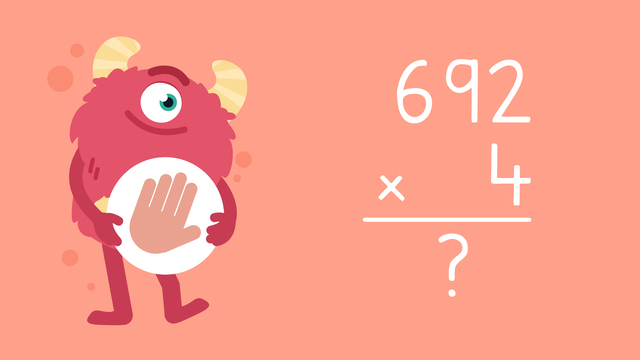# Multiplying up to Three Digits Using the Standard Algorithm—Let's Practice!Rating

Ø 3.7 / 3 ratings
The authorsTeam Digital

## Basics on the topicMultiplying up to Three Digits Using the Standard Algorithm—Let's Practice!

Today we are multiplying up to three digits using the standard algorithm with Razzi! This video contains examples to help you further practice and grow confident in this topic.

### TranscriptMultiplying up to Three Digits Using the Standard Algorithm—Let's Practice!

Razzi says get these items ready (...) Because today we're going to practice... Multiplying up to Three Digits Using the Standard Algorithm. It's time to begin! Solve each multiplication equation using the standard algorithm. Starting with, thirty-eight times eight. Pause the video to work on the problem (...) and press play when you are ready to see the solution! Eight times eight is (...) sixty-four... so put four in the ones place, and regroup the six HERE. Eight times three is (...) twenty-four. Last, add twenty-four and six which equals thirty. Did you also get three hundred four? Let's try one more! Solve six hundred ninety-two times four. Pause the video to work on the problem (...) and press play when you are ready to see the solution! Four times two equals (...) eight... Four times nine equals (...) thirty-six... so put the six in the TENS place, and regroup the three HERE. Four times six equals (...) twenty-four. Last, add twenty-four and three which equals (...) twenty-seven. Did you also get two thousand seven hundred sixty-eight? Razzi had so much fun practicing with you today! See you next time!

1 comment
1 comment
1.This is a interesting :)

From Aaliyah, 13 days ago